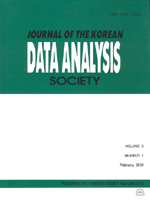상세검색
최근 검색어 전체 삭제
다국어입력
즐겨찾기0KCI등재 학술저널

# The Probability Range of Confidence Intervals for Variance A of Total Variance in the Two-Factor Nested Variance Component Model

• 등재여부 : KCI등재
• 2001.09
• 237 - 243 (7 pages)

The confidence intervals for variance A of the ratio of total variance in the two-factor nested variance component model with equal numbers in the cells is given by various forms by many authors. This article shows that one of these forms is probability ranges of Broemeling s confidence intervals for variance A of total variance in the two-factor nested variance component model.

1. Introduction

2. Variance Components Model

3. The Range of Confidence Intervals

References